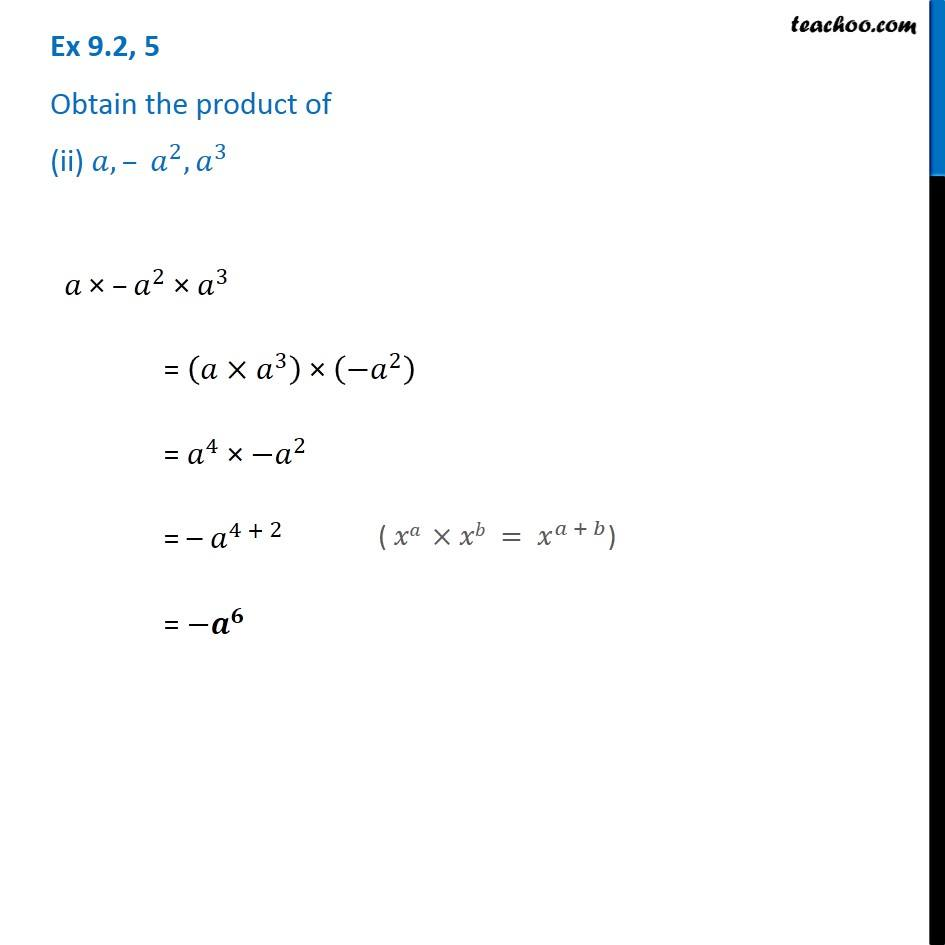Ex 9.2

Chapter 9 Class 8 Algebraic Expressions and Identities
Serial order wiseGet live Maths 1-on-1 Classs - Class 6 to 12

### Transcript

Ex 9.2, 5 Obtain the product of (ii) 𝑎, – 𝑎^2, 𝑎^3 𝑎" ×" –𝑎^2 "× " 𝑎^3 = (𝑎×𝑎^3 ) × (−𝑎^2 ) = 𝑎^4 × 〖−𝑎〗^2 = – 𝑎^(4 + 2) = −𝒂^𝟔 ( 𝑥𝑎 ×𝑥𝑏 = 𝑥^(𝑎 + 𝑏))